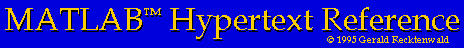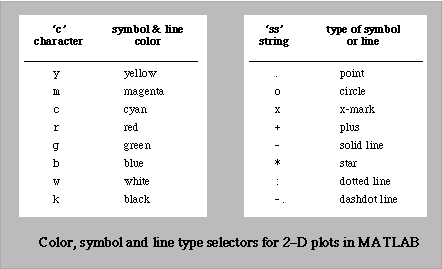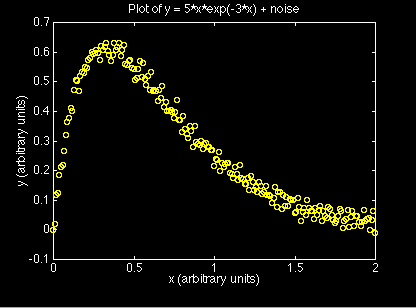# Creating Symbol Plots with MATLAB

This page describes how to plot y = f(x) by putting a symbol at each data point.

## Changing symbol or line types

The symbol or line type for the data can by changed by passing an optional third argument to the plot command. For example

```	>> plot(x,y,'o');
```
plots data in the x and y vectors using circles drawn in the default color (yellow), and
```	>> plot(x,y,'r:');
```
plots data in the x and y vectors by connecting each pair of points with a red dashed line.

The third argument of the plot command is a one, two or three character string of the form 'cs', where 'c' is a single character indicating the color and 's' is a one or two character string indicating the type of symbol or line. The color selection is optional. Allowable color and symbols types are summarized in the following tables. Refer to ``help plot'' for further information.### A simple symbol plot

This example shows you how to plot data with symbols. This type of plot is appropriate, for example, when connecting data points with straight lines would give the misleading impression that the function being plotted is continuous between the sampled data points.

Here are the MATLAB commands to create a symbol plot with the data generated by adding noise to a known function. These commands are also in the script file noisyData.m for you to download.

```	>> x = 0:0.01:2;                 %  generate the x-vector
>> noise = 0.02*randn(size(x));  %  and noise
>> y = 5*x.*exp(-3*x) + noise;   %  Add noise to known function
>> plot(x,y,'o');                %  and plot with symbols
>>
>> xlabel('x (arbitrary units)');          %  add axis labels and plot title
>> ylabel('y (arbitrary units)');
>> title('Plot of y = 5*x*exp(-3*x) + noise');
```
Note that the ``.*'' operator is necessary when multiplying the vector `x` by the vector `exp(-3*x)`. The preceding statments create the following plot.